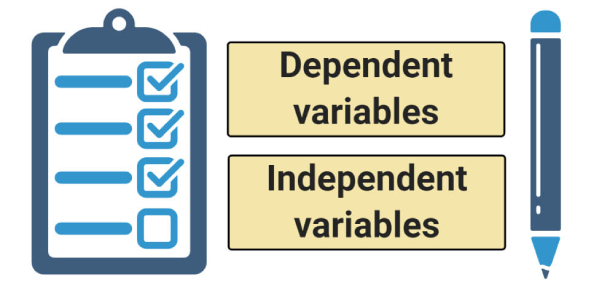# Independent And Dependent Variables Quiz

10 Questions | Attempts: 87643
ShareSettingsWhat do you understand by independent and dependent variables? Here, we present to you the best quiz that will help you to test your knowledge. There are two types of experiments when it comes to any experiment. The independent and dependent variables vary depending on which is being tested in the experiment and which one is controlled in the experiment. By taking the quiz below, test out how well you understand these two types of variables. Start the quiz, then.

• 1.
This variable MUST be measurable.
• A.

Independent Variable (IV)

• B.

Dependent Variable (DV)

• C.

Controlled Variable

• D.

All of these

• 2.
The independent variable (IV) changes the dependent variable (DV).
• A.

True

• B.

False

• 3.
The deeper I cut, the more it will bleed. The 2 variables: 1. Depth of cut 2. Level of bleeding Which is the independent variable?
• A.

Level of bleeding

• B.

Depth of cut

• C.

Both A and B

• D.

None of these

• 4.
If I add more milk, the tea will get a lighter color. The two variables: 1. Amount of milk 2. Color of the tea Which one is the independent variable?
• A.

Amount of milk

• B.

Color of the tea

• C.

Both A and B

• D.

None of these

• 5.
I need to pay a fine because I crossed the red light. The 2 variables: 1. Paying fine 2. Crossing red light Which is the independent variable?
• A.

Crossing red light

• B.

Paying fine

• C.

Both A and B

• D.

None of these

• 6.
Add extra sugar to make it sweeter. Which of these is the dependent variable in this?
• A.

Amount of sugar

• B.

Level of sweetness

• C.

Both A and B

• D.

None of these

• 7.
I will feel lazy if I eat too much. The 2 variables: 1. Amount of food eaten. 2. Level of laziness Which one is the dependent variable?
• A.

Amount of food eaten

• B.

Level of laziness

• C.

Both A and B

• D.

None of these

• 8.
I will lose extra fat If I run extra hours. The 2 variables: 1. Amount of fat lost 2. Hours of running Which is the dependent variable?
• A.

Amount of fat lost

• B.

Hours of running

• C.

Both A and B

• D.

None of these

• 9.
It will get colder if winds blow faster. The 2 variables: 1. Wind's speed 2. Amount of cold Which is the dependent variable?
• A.

Wind's speed

• B.

Amount of cold

• C.

Both A and B

• D.

None of these

• 10.
Does working extra hours in the office get you more recognition? The 2 variables 1. Number of hours 2. Level of recognition Which is the dependent variable?
• A.

Level of recognition

• B.

Number of hours

• C.

Both A and B

• D.

None of these

## Related TopicsBack to top
×

Wait!
Here's an interesting quiz for you.